# Unit Cube - Definition with Examples

The Complete K-5 Math Learning Program Built for Your Child

• 30 Million Kids

Loved by kids and parent worldwide

• 50,000 Schools

Trusted by teachers across schools

• Comprehensive Curriculum

Aligned to Common Core

## Unit Cube Games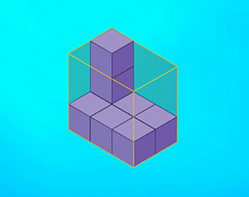Volume using Unit Cubes

A unit cube is a cube which has a volume or capacity of 1 units. You can count the number of unit cubes that can fit into a solid to find its volume.

Covers Common Core Curriculum 5.MD.5.a

## What is Unit Cube?

In geometry, a unit cube is a cube whose each side is 1 unit long.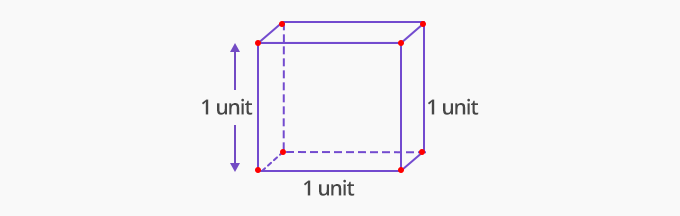## Volume

The amount of space something occupies is called its volume.

Volume of a unit cube:

A cube has all its sides of the same length. A unit cube has all its sides of length 1 unit. So, thevolume of a unit cube = Side × Side × Side

= 1unit × 1unit × 1unit

= 1 cubic unit

The volume of a unit cube is one cubic unit.

If a solid shape is made using unit cubes, then the volume of the shape is equal to the number of unit cubes that make up the shape.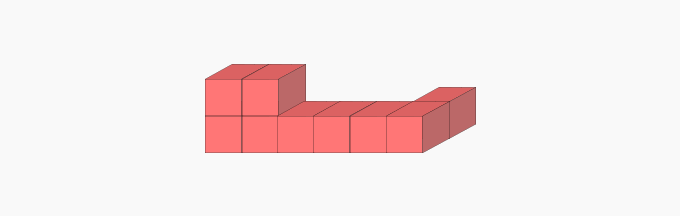This solid is made of 6 unit cubes.

Therefore, its volume is 6 cubic units.

## Surface Area

The total area that the surface of a 3-dimensional object would cover if its sides were laid flat is called it's surface area. Surface area is measured in square units.

Surface Area of a Unit Cube

A cube has 6 square faces. A unit cube has 6 square faces with each side 1 unit long. So, the area of each side of the cube = 1 unit × 1 unit = 1 square unit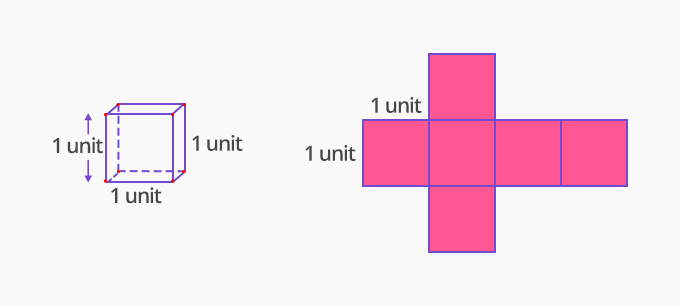As all 6 faces of a cube have the same area,

The surface area of a unit cube = 6 × area of one face

= 6 × 1 square unit

= 6 square units

Therefore, the surface area of a unit cube is 6 square units.

 Fun Facts A unit cube can be used to measure the volume of other objects or solids. To find the volume of objects using unit cubes,  fill the object with unit cubes.  The number of unit cubes that fit in the object is the volume of the object.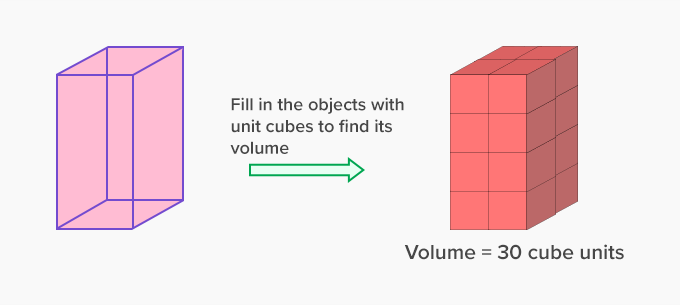Won Numerous Awards & Honors